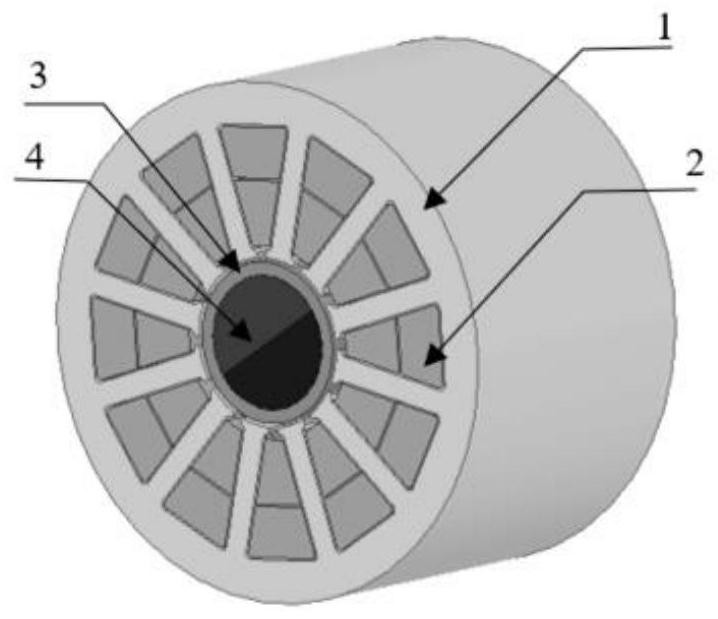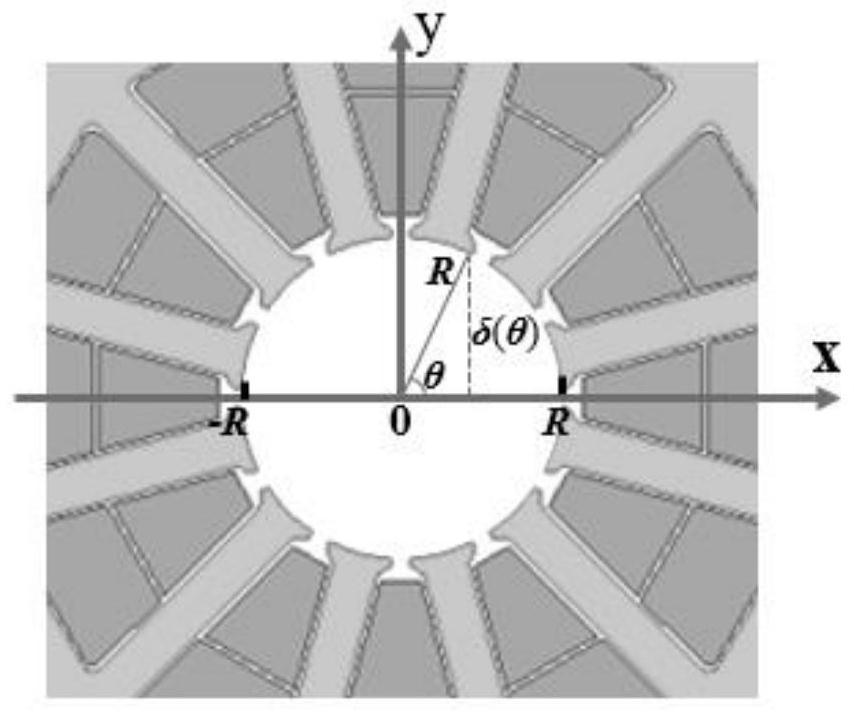# Two-pole permanent magnet solid rotor motor inductance design method based on flux linkage integration method

## A technology of solid rotor and integral method, which is applied in electromechanical devices, manufacturing motor generators, design optimization/simulation, etc., can solve problems such as not being universal, not applicable, multi-computer hardware resources and time, etc. Adaptability requirements, the effect of meeting the rapidity requirements

Pending Publication Date: 2021-02-23
TIANJIN UNIV
6 Cites 2 Cited by

## AI-Extracted Technical Summary

### Problems solved by technology

Since there is no shaft in the two-pole solid permanent magnet rotor, the equivalent air gap lengths at different positions of the rotor are different. The air gap length involved in the traditional empirical coefficient method is not applicable to this type of motor; , it is usually necessary to ca...
View more

## Abstract

The invention provides a two-pole permanent magnet solid rotor motor inductance design method based on a flux linkage integration method. The method comprises the following steps: (1) determining an armature magnetomotive force distribution function; (2) determining an equivalent air gap length function and a magnetic conductance distribution function; (3) calculating the flux linkage of each infinitesimal linkage; and (4) integrating the flux linkage within the inner diameter range of the stator core, calculating the flux linkage of each phase winding of the motor, and obtaining the inductance by using the method of the ratio of the flux linkage to the armature current amplitude.

Application Domain

Design optimisation/simulationManufacturing stator/rotor bodies +1

Technology Topic

MagnetMagnetomotive force +7

## Image

•••## Examples

• Experimental program(1)

### Example Embodiment

In order to make the objects, technical solutions, and advantages of the present invention, it will be described in connection with the examples and the accompanying drawings, and the schematic embodiments of the present invention and their description thereof are for explaining the present invention. As a result of the present invention.
Seefigure 1 The present invention relates to the two-pole permanent magnet solid rotor high-speed permanent magnet motor stator core 1 and the stator winding 2, and the rotor sheath 3 and the rotor magnet 4, the entire system adopts the 2-pole 12 slot, the stator winding adopts a double-layer short-distance distribution Arrangement, the stator windings are divided into three phases, and see the division of the same.figure 2 , Along the counterclockwise direction + a, -C, + b, -a, + c, -b.
The present invention based on magneto-chain branch-based polar permanent magnet solid rotor high speed motor inductive design method, including the following steps:
(1) Determine the armature magnetic distribution function
According to the motor windings theory, the armature magnetic mobility distribution function is: for a particular winding type, the armature magnetic mobility distribution function is:

In the formula, P is the motor extreme logarithm, and n is a series series, i is a raw value of the armature current, kDP For a phased winding coefficient.
For the two-pole permanent magnet solid rotor high speed motor, its extreme logarithm P = 1, the electromagnetic angle is the same, so the armature magnetic distribution function f (θ) is:

(2) Determine equivalent air gap length function and magnetic distribution function
The relative magnetic guidance of the rotor magnet and the rotor sheath is close to the air, and it can be ignored when calculating the equivalent air gap length.image 3The value of the equivalent gas gap length is known that when θ is 0 ° and 180 °, the air gap is equivalent to 0, and is a minimum value; when θ is 90 °, the air gap is equivalent length is R, for the maximum value, the equivalent gas gap length at each θ is different, and the equivalent air gap length at different positions is:
δ (θ) = R sin (θ) (3)
In the formula, R represents the inner surface radius of the stator core, and the relationship between the magnetic guidance and the equivalent air gap length in the motor is known that the magnetic distribution function on the unit axial length is:

In μ0Vacuum magnetic permeability.
(3) Calculate the magnetic chain of each micro-cell line:
It can be seen from (2) and (4), the magnetic guidance of the armature magnetic movement and the unit axial length is a function of θ, and the two are multiplied by the macromavern, and the two are distributed in space when the stator core magnetic presses. Function, and considering tiny distance increment DX, each micro-rational magnetic chain is:
dψm1= NKDP lEF F (θ) λ (θ) DX (5)
In LEF The axial length of the motor.
(4) Integrating the magnetic chain within the inner diameter range of the stator core, calculating the magnetic chain of each phase of the motor, and the method of obtaining the ratio of the magnetic chain and the armature gner value:

The (2) ~ (5) is substituted (6), and the final analysis of the inductance can be obtained.

In the present invention, under the premise of the counter-core magnetic pressure drop, it is determined based on the electromagnetic momentum and the magnetic distribution function, and the magnetature magnetic guidance distribution function is considered. With magnetic chain branch, the ratio of the magnetic chain and current is used to supply an inductor to provide a reliable design method for the polar permanent magnet solid rotor high-speed motor inductance.

## PUM## Description & Claims & Application Information

We can also present the details of the Description, Claims and Application information to help users get a comprehensive understanding of the technical details of the patent, such as background art, summary of invention, brief description of drawings, description of embodiments, and other original content. On the other hand, users can also determine the specific scope of protection of the technology through the list of claims; as well as understand the changes in the life cycle of the technology with the presentation of the patent timeline. Login to view more.
Who we serve
• R&D Engineer
• R&D Manager
• IP Professional
Why Eureka